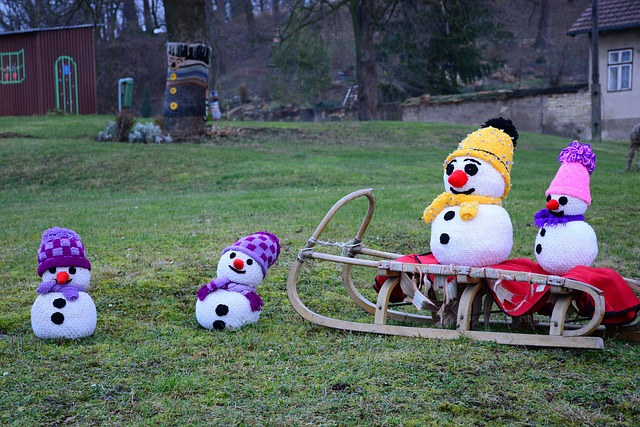#### You may also like### Curvy Equation

This problem asks you to use your curve sketching knowledge to find all the solutions to an equation.### Digital Equation

Can you find a three digit number which is equal to the sum of the hundreds digit, the square of the tens digit and the cube of the units digit?### Euler's Totient Function

How many numbers are there less than $n$ which have no common factors with $n$?

# Frosty the Snowman

##### Age 16 to 18Challenge LevelIf you are getting stuck on this question, start by reading A Guide to Problem Solving.  Also try taking a break (take the dog/chicken for a walk, have some diner, sleep on it!) and then try again.

You can also use the Getting Started tab, where you will find some hints and tips to guide you through the question.

Frosty the snowman is made from two uniform spherical snowballs, of initial radii $2R$ and $3R.$ The smaller (which is his head) stands on top of the larger.

As each snowball melts, its volume decreases at a rate which is directly proportional to its surface area, the constant of proportionality being the same for both snowballs. During melting each snowball remains spherical and uniform.

• When Frosty is half his initial height, show that the ratio of his volume to his initial volume is 37 : 224 .

• What is this ratio when Frosty is one-tenth of his initial height?

Are the assumptions in this question reasonable?  In what ways might the question be modified to make it more realistic?

Can you think of any other questions to ask about Frosty's plight?

Frosty the snowman appears again in A Frosty Puddle.

Adapted from STEP Mathematics I, 1991, Q2. Question reproduced by kind permission of Cambridge Assessment Group Archives. The question remains Copyright University of Cambridge Local Examinations Syndicate ("UCLES"), All rights reserved.